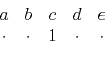Spatial aliasing and scale invarianceNext: MULTISCALE, SELF-SIMILAR FITTING Up: INTERPOLATION BEYOND ALIASING Previous: INTERPOLATION BEYOND ALIASING

Interlacing a filter

The filter below can be designed despite alternate missing traces. This filter destroys plane waves. If the plane wave should happen to pass halfway between the d'' and the e'', those two points could interpolate the halfway point, at least for well-sampled temporal frequencies, and the time axis should always be well sampled. For example,would almost destroy the plane wave and it is an aliased planewave for its higher frequencies.(1)

We could use module pefto find the filter (1), if we set up the lag table lag appropriately. Then we could throw away alternate zeroed rows and columns (rescale the lag) to get the filter(2)

which could be used with subroutine mis1(), to find the interleaved data because both the filters (1) and (2) have the same dip characteristics.

Figure 1 shows three plane waves recorded on five channels and the interpolated data.lace3
Figure 1.
Left is five signals, each showing three arrivals. With the data shown on the left (and no more), the signals have been interpolated. Three new traces appear between each given trace, as shown on the right.Both the original data and the interpolated data can be described as beyond aliasing,'' because on the input data the signal shifts exceed the signal duration. The calculation requires only a few seconds of a two-stage least-squares method, in which the first stage estimates a PEF (inverse spectrum) of the known data, and the second uses the PEF to estimate the missing traces. Figure 1 comes from PVI which introduces the clever method described above. We will review how that was done and examine the F90 codes that generalize it to-dimensions. Then we'll go on to more general methods that allow missing data in any location. Before the methods of this section are applied to field data for migration, data must be broken into many overlapping tiles of size about like those shown here and the results from each tile pieced together. That is described later in chapter.

A PEF is like a differential equation. The more plane-wave solutions you expect, the more lags you need on the data. Returning to Figure 1, the filter must cover four traces (or more) to enable it to predict three plane waves. In this case, na=(9,4). As usual, the spike on the 2-D PEF is at center=(5,1). We see the filter is expanded by a factor of jump=4. The data size is nd=(75,5) and gap=0. Before looking at the code lacefor estimating the PEF, it might be helpful to recall the basic utilities line2cart and cart2linefor conversion between a multidimensional space and the helix filter lag. We need to sweep across the whole filter and stretch'' its lags on the 1-axis. We do not need to stretch its lags on the 2-axis because the data has not yet been interlaced by zero traces.

user/gee/lace.c
 sf_filter lace_pef(int dim /* number of dimensions */, float *dd /* data */, int jump /* filter stretch */, int n /* data size */, int *nd /* data dimensions [dim] */, int *center /* filter center [dim] */, int *gap /* filter gap [dim] */, int *na /* filter size [dim] */) /*< estimate PEF >*/ { int *savelags, ii[SF_MAX_DIM]; /* holding place */ int ih, nh, lag0, j; sf_filter aa; aa = createhelix(dim, nd, center, gap, na); savelags = aa->lag; nh = aa->nh; aa->lag = sf_intalloc(nh); /* prepare interlaced helix */ lag0 = sf_cart2line(dim, na, center); for (ih=0; ih < nh; ih++) { /* sweep through the filter */ sf_line2cart(dim, na, ih+lag0+1, ii); for (j=0; j < dim; j++) { ii[j] -= center[j]; } ii *= jump; /* interlace on 1-axis */ aa->lag[ih] = sf_cart2line(dim, nd, ii); } na *= jump; bound(dim, nd, nd, na, aa); /* define aa->mis */ na /= jump; find_pef(n, dd, aa, nh*2); /* estimate aa coefficients */ free(aa->lag); aa->lag = savelags; /* restore filter lags */ return aa; }
The line ii *= jump means we interlace the 1-axis but not the 2-axis because the data has not yet been interlaced with zero traces.

After the PEF has been found, we can get missing data in the usual way with with module mis2.Spatial aliasing and scale invarianceNext: MULTISCALE, SELF-SIMILAR FITTING Up: INTERPOLATION BEYOND ALIASING Previous: INTERPOLATION BEYOND ALIASING

2013-07-26